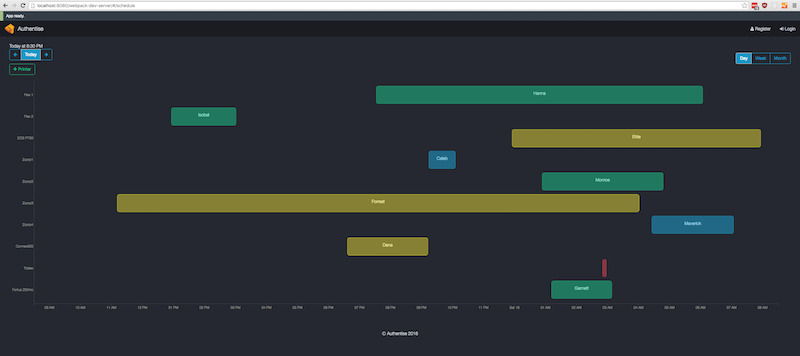# Gantt chart with React.js and D3.jsLast week I had to create a [Gantt chart] that is showing prints over time. I couldn’t find a React.js component of a [Gantt chart], instead I found some Timeline components but after sinking too much time into bugs and inability to customize them, I decided to write on myself. I had to decide between using canvas or svg. There are many great charting libraries out there but I always wanted to give D3.js a try.

My experience with D3.js starting guide was not smooth and I don’t recommend it to others who are starting with D3.js. Instead, I looked into the source of the D3.js examples here: https://github.com/d3/d3/wiki/Gallery. Using this approach I had the chart in no time.

Next, I wanted to create a React.js component that I could reuse in my application. I decided to initialize D3.js inside `componentDidMount` because it is invoked once, immediately after the initial rendering occurs. Here is what my componentDidMount looks like:

```componentDidMount() {
const {start_date, end_date, modelers, builds, view} = this.props

const svg = d3.select('svg.gantt')
const width = svg.node().parentNode.offsetWidth - (MARGIN.left + MARGIN.right)
const height = 800 - (MARGIN.top + MARGIN.bottom)

const x = d3.time.scale().domain([start_date, end_date]).range([0, width]).clamp(true)
const y = d3.scale.ordinal().domain(modelers).rangeRoundBands([0, height - (MARGIN.top + MARGIN.bottom)], 0.2)
const x_axis = d3.svg.axis().scale(x).ticks(this.getTicksInterval(view), this.getTicksCount(view, width)).tickSubdivide(true)
const y_axis = d3.svg.axis().scale(y).orient('left').tickSize(0)

svg.attr('viewBox', `0 0 \${width + MARGIN.left + MARGIN.right} \${height + MARGIN.top + MARGIN.bottom}`)

const chart = svg.append("g").attr('class', 'chart-holder').attr('transform', `translate(\${MARGIN.left}, \${MARGIN.top})`)

chart
.append("g")
.attr('class', 'x axis')
.attr("transform", "translate(0,"+(height - MARGIN.top - MARGIN.bottom)+")")
.call(x_axis);

chart
.append("g")
.attr('class', 'y axis')
.call(y_axis);
}
```

Whenever my state is changing, I’d use `componentDidUpdate` to update the chart. In this method I am redrawing the axis:

```const x = d3.time.scale().domain([start_date, end_date]).range([0, width]).clamp(true)
const y = d3.scale.ordinal().domain(modelers).rangeRoundBands([0, height - (MARGIN.top + MARGIN.bottom)], 0.2)
const x_axis = d3.svg.axis().scale(x).ticks(this.getTicksInterval(view), this.getTicksCount(view, width))
const y_axis = d3.svg.axis().scale(y).orient('left').tickSize(0)
d3.select('g.x.axis').transition().call(x_axis)
d3.select('g.y.axis').transition().call(y_axis)
```

and the chart data:

```const _builds = d3.select('g.chart-holder').selectAll('g.build-container').data(builds, (build) => build.uri)

const build = _builds.enter()
.append('g')
.attr('class', 'build-container')

_builds.exit().remove()
```

D3.js syntax reminds me close to that of jQuery. It was not difficult to pick it up. Looking up documentation of functions is easy and I am pleased with how detailed the wiki is. I recommend using D3.js for your next charting project.TIP: if you are using webpack development server with hot reloading, you will need to use process.nextTick in componentDidMount to initialize D3.js.